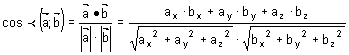# How is the scalar multiplication defined

The scalar product of two vectors Andreas Pester University of Applied Sciences Techikum Kärnten, Villach
[email protected]Summary: In this section the concept of the scalar product of two vectors is explained

Keywords: Definition | Properties of the scalar product

The scalar product of two vectors is the multiplication of the projection of the vector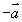on the vector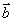with the amount ofThe scalar product of two vectors gives a scalar quantity and is defined by: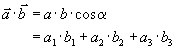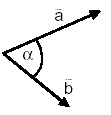Where a is the angle between the two vectorsand.

An example of this is: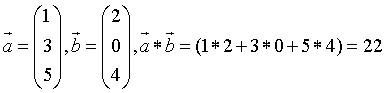As you can see, the result is a number (22), not a vector.

The following applies to the scalar product (if it can be calculated at all):

 1)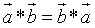Commutative law 2)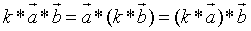Distributive law 3)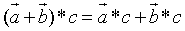Associative law

#### Orthogonal vectors

Two vectors different from the zero vector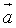andstand exactly then perpendicular on each other, so are orthogonalif its scalar product vanishes: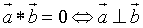.

#### Application of the scalar product for the angle calculation: